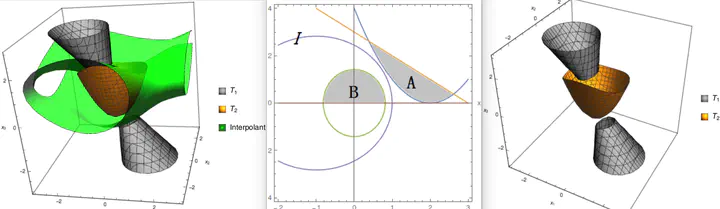# Interpolant Synthesis for Quadratic Polynomial Inequalities and Combination with EUF### Abstract

An algorithm for generating interpolants for formulas which are conjunctions of quadratic polynomial inequalities (both strict and nonstrict) is proposed. The algorithm is based on a key observation that quadratic polynomial inequalities can be linearized if they are concave. A generalization of Motzkin’s transposition theorem is proved, which is used to generate an interpolant between two mutually contradictory conjunctions of polynomial inequalities, using semi-definite programming in time complexity $\mathcal{O}(n^3+nm)$, where $n$ is the number of variables and $m$ is the number of inequalities (this complexity analysis assumes that despite the numerical nature of approximate SDP algorithms, they are able to generate correct answers in a fixed number of calls). Using the framework proposed by Sofronie-Stokkermans for combining interpolants for a combination of quantifier-free theories which have their own interpolation algorithms, a combination algorithm is given for the combined theory of concave quadratic polynomial inequalities and the equality theory over uninterpreted functions.

Type
Publication
In IJCAR 2016##### Mingshuai Chen
###### ZJU100 Young Professor

My research interests include formal verification, programming theory, and logical aspects of computer science.